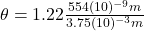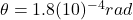## The limit to the eye’s acuity is actually related to diffraction by the pupil. What is the angle between two just‑resolvable points of light

Question

The limit to the eye’s acuity is actually related to diffraction by the pupil. What is the angle between two just‑resolvable points of light for a 3.75 mm 3.75 mm diameter pupil, assuming the average wavelength of 554 nm 554 nm ?

in progress 0
2 months 2021-07-31T18:52:53+00:00 1 Answers 4 views 0

1. Answer: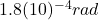Explanation:

This problem is related to the Rayleigh Criterion, which provides the following formula to find the acuity or limit of resolution of an optic system with circular aperture (the eye in this case):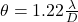Where: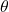is the angle of resolution (related to the acuity)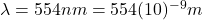is the wavelength of the light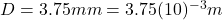is the diameter of the pupil

Solving: